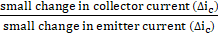# The current gain of a transistor in the common base mode is 0.9. If the change in the emitter current is 5 mA, the change in the collector current will be a) 4 mA b) 4.5 mA c) 5.6 mA d) Zero

## Question ID - 151128 :- The current gain of a transistor in the common base mode is 0.9. If the change in the emitter current is 5 mA, the change in the collector current will be a) 4 mA b) 4.5 mA c) 5.6 mA d) Zero

3537

 (b) Current gain =0.9 == 0.95 = 4.5 mA

Next Question :

A wire of lengthand 3 identical cells of negligible internal resistances are connected in series. Due to the current, the temperature of the wire due to the current, the temperature of the wire is raised byis a time. A numberof similar cells is now connected in series with a wire of the same material and cross-section but of length. The temperature of the wire is raised by the same amountin the same time. The value ofis